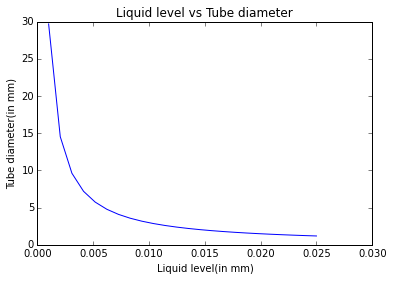# Chapter3-Fluid Statics¶

## Ex1-pg58¶

In :
#calculate the tube diameter and mercury meter level
import numpy
%matplotlib inline

import warnings
warnings.filterwarnings('ignore')
import math
import matplotlib
from matplotlib import pyplot
##Surface tension of water(in mN/m):
STw=72.8*10**-3;
##Surface Tension of mercury(in mN/m):
STm=375*10**-3;
##Contact angle for water:
thetaw=0.;
##COntact angle for mercury:
thetam=140.;
##Density of water(in kg/m**3):
dw=1.;
##Density of mercury(in kg/m**3):
dm=13.6;
##Acceleration de to gravity(in m/sec):
g=9.81;
##liquid level##

##Tube diameter(in mm):
D =numpy.linspace(1,25,num=24)
D1=D/1000.
n=len(D1)
dhw=numpy.zeros(n)
dhm=numpy.zeros(n)
for i in range (0,n):
##Change in liquid level for water(in mm):
dhw[i]=4*STw*math.cos(thetaw/57.3)/dw/g/D1[i];
##Change in liquid level for mercury(in mm):
dhm[i]=4*STm*math.cos(thetam/57.3)/dm/g/D1[i];

print D1
##Plotting tube daimeter and water level:
pyplot.plot(D1,dhw)
##Plotting tube daimeter and mercury level:

pyplot.xlabel('Liquid level(in mm)')
pyplot.ylabel('Tube diameter(in mm)')
pyplot.title('Liquid level vs Tube diameter')
pyplot.show()

[ 0.001       0.00204348  0.00308696  0.00413043  0.00517391  0.00621739
0.00726087  0.00830435  0.00934783  0.0103913   0.01143478  0.01247826
0.01352174  0.01456522  0.0156087   0.01665217  0.01769565  0.01873913
0.01978261  0.02082609  0.02186957  0.02291304  0.02395652  0.025     ]## Ex3-pg62¶

In :
##Acceleration due to gravity(in ft/sec**2):
#calculate the Pressure difference between A and B
g=32.2;
##Specific gravity of mercury:
SGm=13.6;
##Specific gravity of oil:
SGo=0.88;
##Specific gravity of water:
SGw=1.;
##Density of water(in slug/ft**3):
d=1.94;
##Heights of liquid in various tubes(in inches):
d1=10.;
d2=3.;
d3=4.;
d4=5.;
d5=8.;
##pressure difference##
##Pressure difference(in lbf/in**2):
dp=g*d*(-d1+SGm*d2-SGo*d3+SGm*d4+d5)/12./144.
print("\n\nRESULTS\n\n")
print'%s %.2f %s'%("\n\nPressure difference between A and B:",dp," lbf/in^2\n\n")


RESULTS

Pressure difference between A and B: 3.73  lbf/in^2



## Ex4-pg64¶

In :
import math
#calculate the If temperature varies linearly with altitude and If density is constant and For an adiabatic atmosphere
##Elevation of Denver(in ft):
z1=5280.;
##Pressure at Denver(in mm of Hg):
p1=24.8;
##Temperature at Denver(in F):
T1=80.;
##Elevation at Vail Pass(in ft):
z2=10600.;
##Temperature at Vsil Pass(in F):
T2=62.;
##Value of R in ft-lbf/lbm-R):
R=53.3;
##Acceleration due togravity(in ft/sec**2):
g=32.2;
k=1.4;

##temperature and pressure##

##Assuming temperature varies linearly with altitude:
m=(T1-T2)/(z2-z1)
##Value of g/(m*R):
v=g/m/R/32.2
##Pressure at Vail Pass(in inches of Hg):
p12=p1*((T2+460.)/(T1+460.))**v
##Percentage change in density:
pc1=(p12/p1*(T1+460.)/(T2+460.)-1.)*100.
##Assuming density is constant:
##Pressure at Vail Pass(in inches of Hg):
p22=p1*(1.-(g*(z2-z1)/(R*32.2)/(T1+460.)))
##Percentage change in density:
pc2=0.;
##Assuming temperature is constant:
##Pressure at Vail Pass(in inches of Hg):
p32=p1*math.e**(-g*(z2-z1)/(R*32.2)/(T2+460.))
##Percentage change in density:
pc3=(p32/p1*(T1+460.)/(T1+460.)-1)*100.
p42=p1*((62.+460.)/(80.+460.))**(k/(k-1.))
##Percentage change in density:
pc4=(p42/p1*(T1+460.)/(T2+460.)-1)*100.
print ("RESULTS")
print ("1) If temperature varies linearly with altitude")
print'%s %.2f %s'% ("\tAtmospheric pressure at Vail Pass: ",p12," inches of Hg")
print'%s %.2f %s'% ("\tPercentage change in density wrt Denver:",pc1," percent")
print("2) If density is constant")
print'%s %.2f %s'% ("\tAtmospheric pressure at Vail Pass:",p22," inches of Hg")
print'%s %.2f %s'% ("\tPercentage change in density wrt Denver: ",pc2," percent")
print("3) If temperature is constant")
print'%s %.2f %s'% ("\tAtmospheric pressure at Vail Pass: ",p32," inches of Hg")
print'%s %.2f %s'% ("\tPercentage change in density wrt Denver:",pc3," percent")
print ("4) For an adiabatic atmosphere")
print'%s %.2f %s'% ("\tAtmospheric pressure at Vail Pass: ",p42," inches of Hg")
print'%s %.2f %s'% ("\tPercentage change in density wrt Denver: ",pc4," percent")
print("due to rounding off error answer is slightly diffeerent")

RESULTS
1) If temperature varies linearly with altitude
Atmospheric pressure at Vail Pass:  20.55  inches of Hg
Percentage change in density wrt Denver: -14.28  percent
2) If density is constant
Atmospheric pressure at Vail Pass: 20.22  inches of Hg
Percentage change in density wrt Denver:  0.00  percent
3) If temperature is constant
Atmospheric pressure at Vail Pass:  20.48  inches of Hg
Percentage change in density wrt Denver: -17.40  percent
Atmospheric pressure at Vail Pass:  22.03  inches of Hg
Percentage change in density wrt Denver:  -8.13  percent
due to rounding off error answer is slightly diffeerent


## Ex5-pg70¶

In :
##Length of gate(in m):
import math
#calculate Net force on the gate and Coordinate of centre of pressure
L=4.;
##Width of gate(in m):
w=5.;
##Depth of gate under water(in m):
D=2.;
##Density of water(in kg/m**3:
d=999.;
##Acceleration due to gravity(in m/sec**2):
g=9.81;
##Angle of gate with horizontal:
theta=30.;
##force and pressure##

##Net force on the gate(in kN):
Fr=d*g*w*(D*L+L**2./2.*math.sin(theta/57.3))
##Centre of pressure:
##Calculation for y coordinate:
yc=D/math.sin(theta/57.3)+L/2. #Area(in m**2):
A=L*w
##Moment of inertia of rectangular gate(in m**4):
Ixx=w*L**3./12.
##y coordinate(in m):
y=yc+Ixx/A/yc
##Calculation for x coordinate:
Ixy=0.
xc=w/2.
##x coordinate(in m):
x=xc+Ixy/A/xc
print("RESULTS")
print'%s %.2f %s'% ("Net force on the gate: ",Fr/1000,"kN")
print'%s %.2f %s %.2f %s '% ("Coordinate of centre of pressure:",x," "and " ",y,"")


RESULTS
Net force on the gate:  588.00 kN
Coordinate of centre of pressure: 2.50   6.22


## Ex6-pg73¶

In :
#Pressure apllied on the door(in psfg):
#calculate the Force requiredto kep the door shut
p0=100.;
import math
import scipy
from scipy import integrate
#Length of door(in feet):
L=3.;
b=2.;
#Density of liqiuid(in lbf/ft^3):
d=100;
#force//
#Force required to keep the door shut(in lbf):
def fun(z):
y=b/L*p0*z+d*b/L*(L*z-z**2)
return y

print("\n\nRESULTS\n\n")
print("\n\nForce requiredto kep the door shut: ",Ft," lbf\n\n")


RESULTS

('\n\nForce requiredto kep the door shut: ', 600.0, ' lbf\n\n')


## Ex7-pg76¶

In :
##Width of gate(in m):
#calculate the orce requiredto kep the door shut and Force required to keep the gate at equilibrium
import math
import scipy
from scipy import integrate
w=5.;
##Depth of water(in m):
D=4.;
##Density of water(in kg/m**3);
d=999.;
##Accelration deto gravity(in m/sec**2):
g=9.81;
##Value of a (in m):
a=4.;
##Point where force acts(in m):
l=5.;
##force at equilibrium##
##Horizontal component of resultant force(in kN):
Frh=0.5*d*g*w*D**2.
##Line of action of Frh(in m):
y1=0.5*D+w*D**3./12./(0.5*D)/(w*D)
##Vertical component of resultant force(in kN):
def fun(x):
y=d*g*w*(D-math.sqrt(a*x))
return y
##Line of acion of Frv(in m):
def fun(x1):
k=d*g*w/Frv*x1*(D-math.sqrt(a*x1))
return k
y=xa
print'%s %.2e %s'%("\n\nForce requiredto kep the door shut: ",y," lbf\n\n")
##Force required to keep the gate in equilibrium(in kN):

Fa=1./l*(xa*Frv+(D-y1)*Frh)
print("RESULTS")
print'%s %.2f %s'%("Force required to keep the gate at equilibrium:  ",Fa/1000,"")


Force requiredto kep the door shut:  1.33e-15  lbf

RESULTS
Force required to keep the gate at equilibrium:   104.54